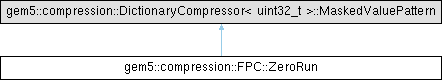gem5  v22.1.0.0
gem5::compression::FPC::ZeroRun Class Reference

`#include <fpc.hh>`

Inheritance diagram for gem5::compression::FPC::ZeroRun:## Public Member Functions

ZeroRun (const DictionaryEntry bytes, const int match_location)

std::size_t getSizeBits () const override

int getRunLength () const
Get the number of zeros in the run so far. More...

void setRunLength (int length)
Set the number of zeros in the run so far. More...

void setRealSize (int size)
When the real size is set it means that we are adding the main zero run pattern. More...

## Private Attributes

int _runLength
Run length so far. More...

int _realSize
A zero run consists of a main ZeroRun pattern, which has a meaningful real size (i.e., different from zero), and X-1 fake (i.e., they are zero-sized, and don't exist in a real implementation) patterns, with X being the size of the zero run. More...

## Detailed Description

Definition at line 156 of file fpc.hh.

## ◆ ZeroRun()

 gem5::compression::FPC::ZeroRun::ZeroRun ( const DictionaryEntry bytes, const int match_location )
inline

Definition at line 171 of file fpc.hh.

## ◆ getRunLength()

 int gem5::compression::FPC::ZeroRun::getRunLength ( ) const
inline

Get the number of zeros in the run so far.

Returns
The number of zeros in this run.

Definition at line 189 of file fpc.hh.

References _runLength.

## ◆ getSizeBits()

 std::size_t gem5::compression::FPC::ZeroRun::getSizeBits ( ) const
inlineoverride

Definition at line 179 of file fpc.hh.

References _realSize.

## ◆ setRealSize()

 void gem5::compression::FPC::ZeroRun::setRealSize ( int size )
inline

When the real size is set it means that we are adding the main zero run pattern.

When that happens, the metadata length must also be taken into account for the size calculation.

Parameters
 size Number of bits used to represent the number of zeros in the run.

Definition at line 206 of file fpc.hh.

References _realSize.

## ◆ setRunLength()

 void gem5::compression::FPC::ZeroRun::setRunLength ( int length )
inline

Set the number of zeros in the run so far.

Parameters
 The number of zeros in this run.

Definition at line 196 of file fpc.hh.

References _runLength.

## ◆ _realSize

 int gem5::compression::FPC::ZeroRun::_realSize
private

A zero run consists of a main ZeroRun pattern, which has a meaningful real size (i.e., different from zero), and X-1 fake (i.e., they are zero-sized, and don't exist in a real implementation) patterns, with X being the size of the zero run.

Definition at line 168 of file fpc.hh.

Referenced by getSizeBits(), and setRealSize().

## ◆ _runLength

 int gem5::compression::FPC::ZeroRun::_runLength
private

Run length so far.

Definition at line 160 of file fpc.hh.

Referenced by getRunLength(), and setRunLength().

The documentation for this class was generated from the following file:
• mem/cache/compressors/fpc.hh

Generated on Wed Dec 21 2022 10:23:39 for gem5 by doxygen 1.9.1